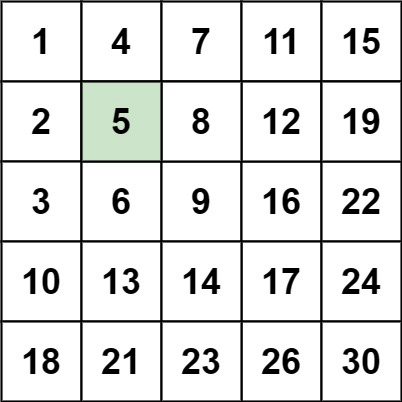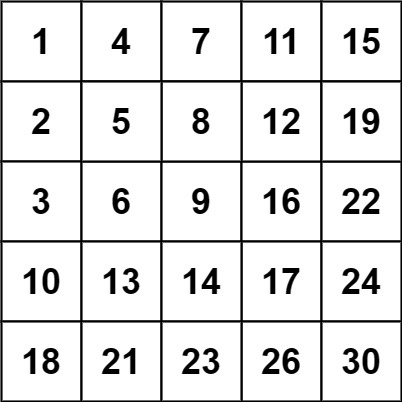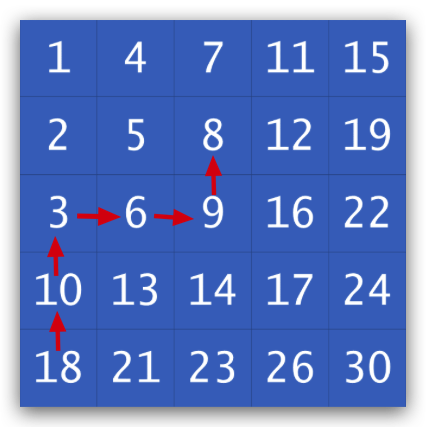240. 搜索二维矩阵 II• m == matrix.length
• n == matrix[i].length
• 1 <= n, m <= 300
• -10^9 <= matix[i][j] <= 10^9
• 每行的所有元素从左到右升序排列
• 每列的所有元素从上到下升序排列
• -10^9 <= target <= 10^9